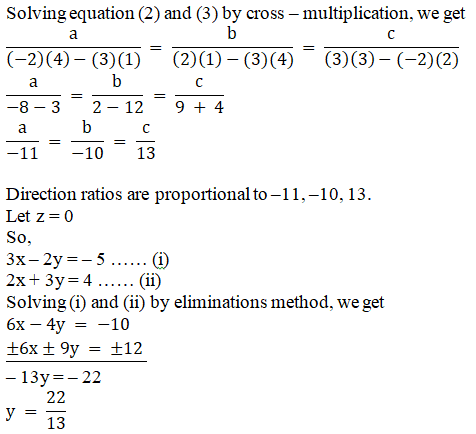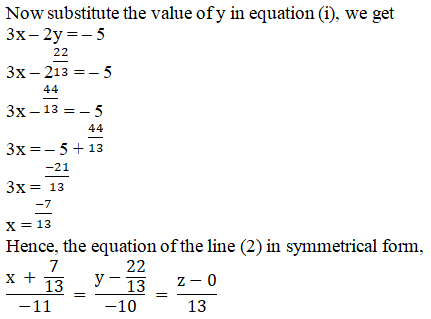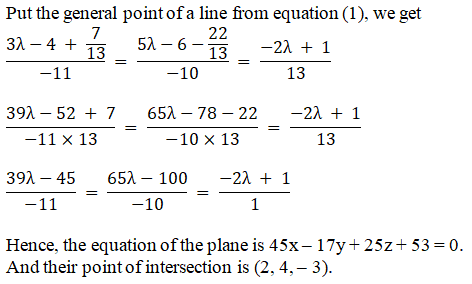# RD Sharma Solutions Class 12 The Plane Exercise 29.13

RD Sharma Solutions for Class 12 Maths Chapter 29 The Plane Exercise 29.13, is provided here. Students who aim to top their board exams can get access to the RD Sharma Solutions for Class 12 and prepare themselves confidently for the final exams. Experts have formulated the solutions in an easily understandable manner for students to grasp the concepts easily and score good marks in the exams. Students can easily download the pdf consisting of this chapter solutions, which are available in the links provided below.

## Download PDF of RD Sharma Solutions For Class 12 Maths Chapter 29 Exercise 13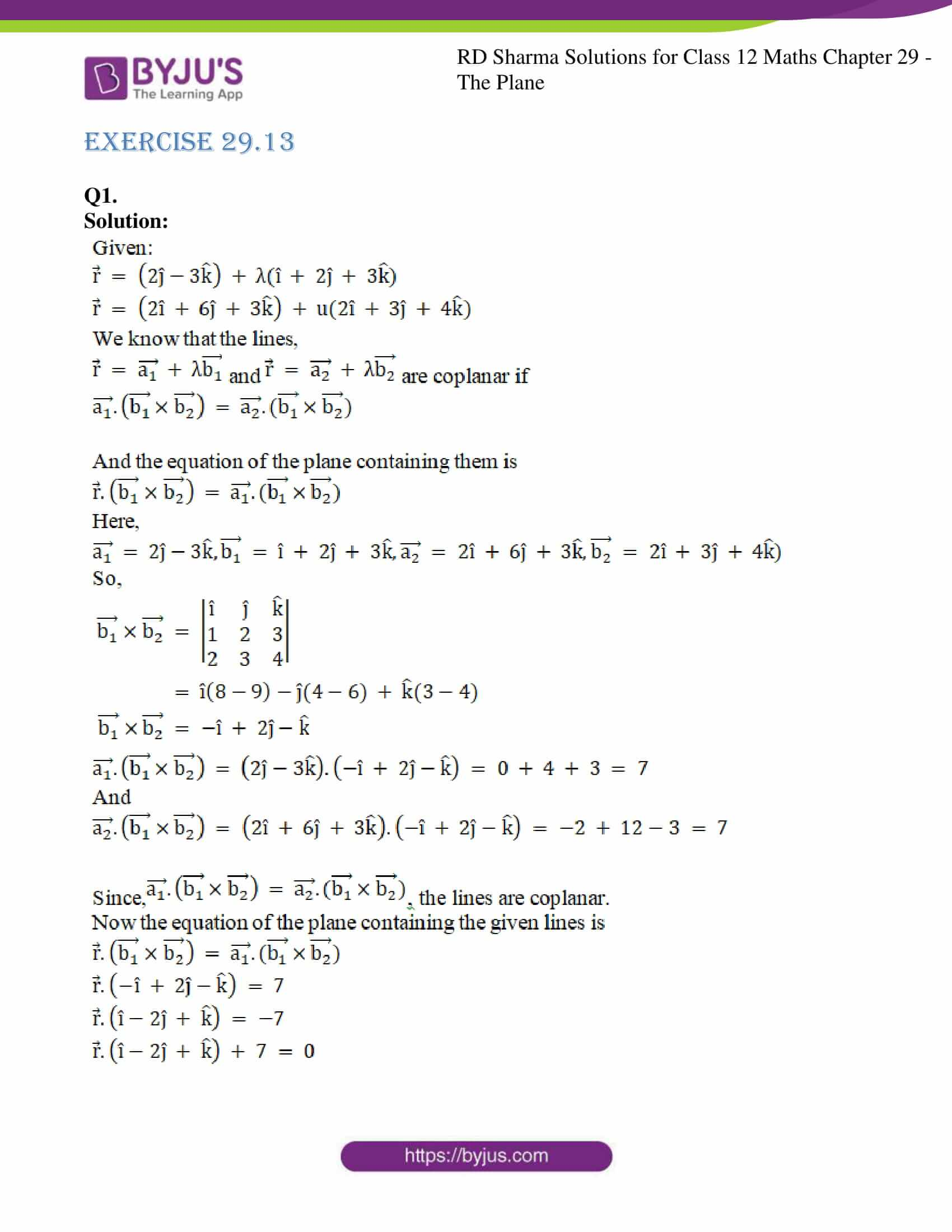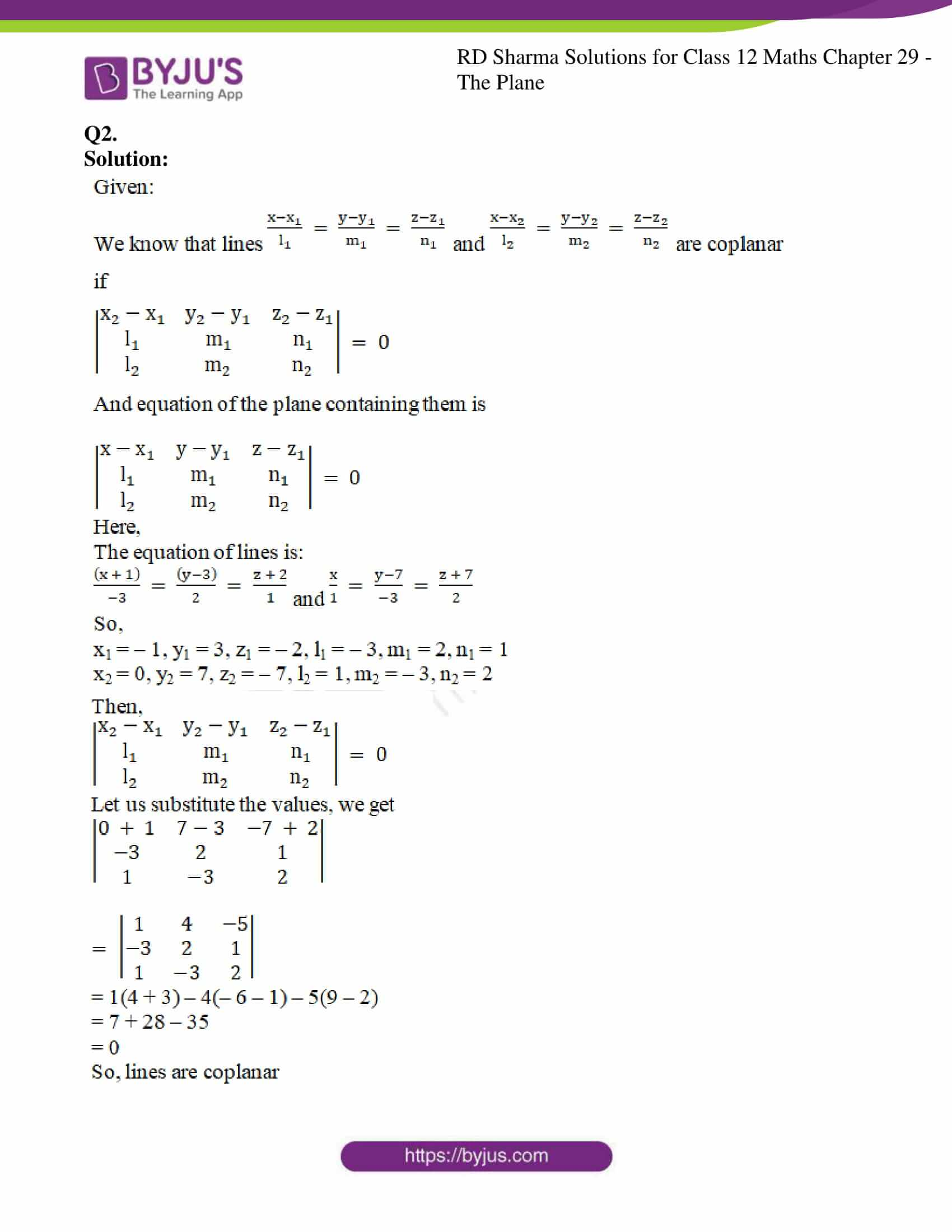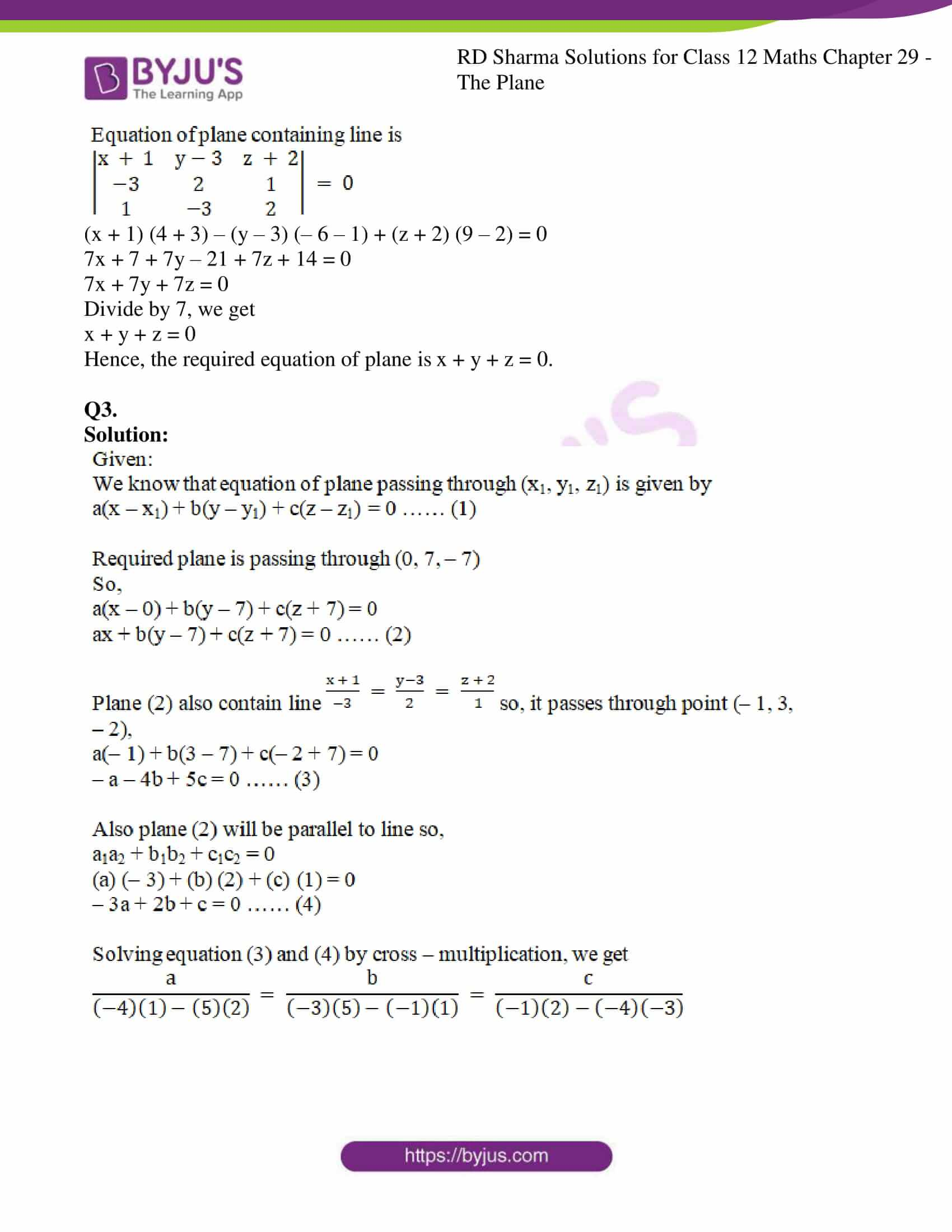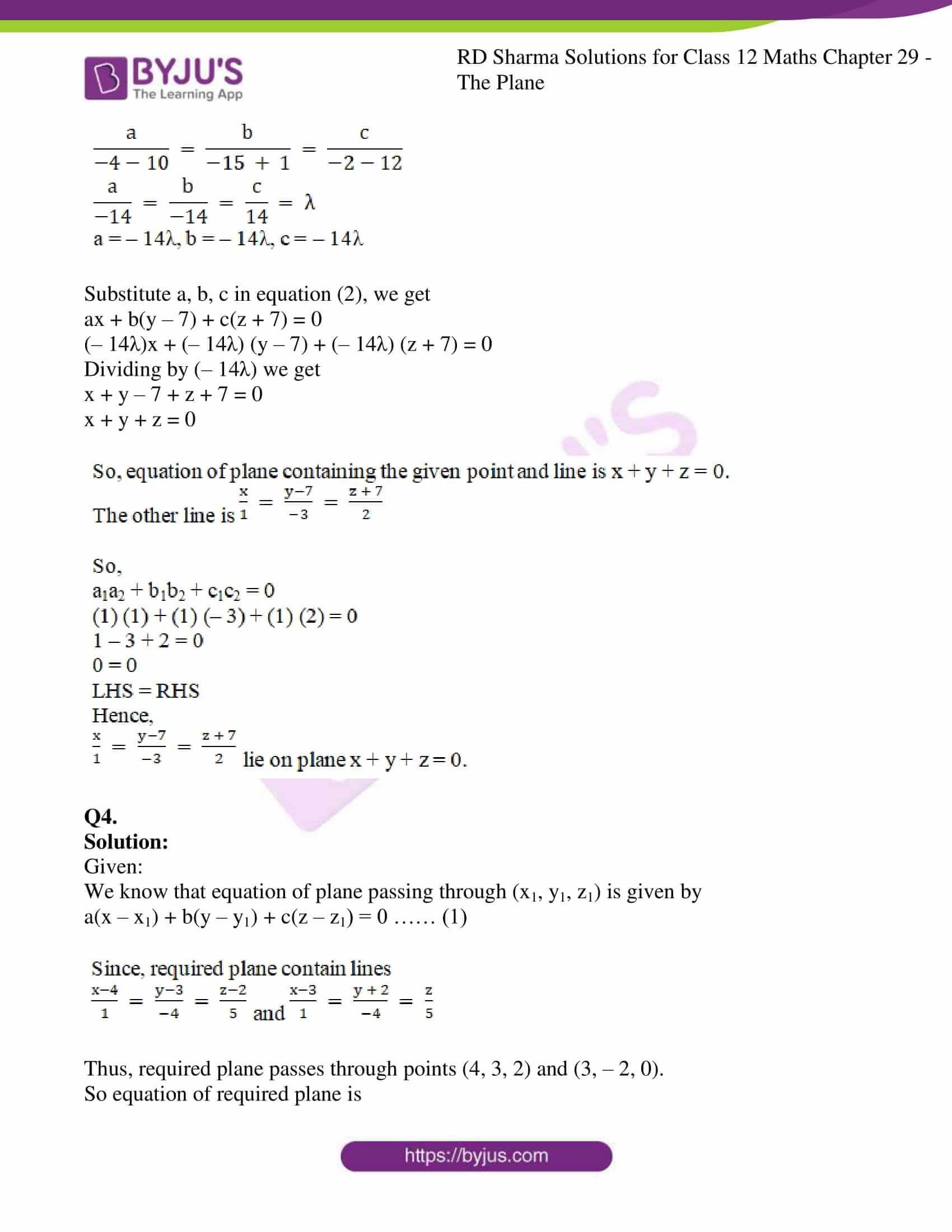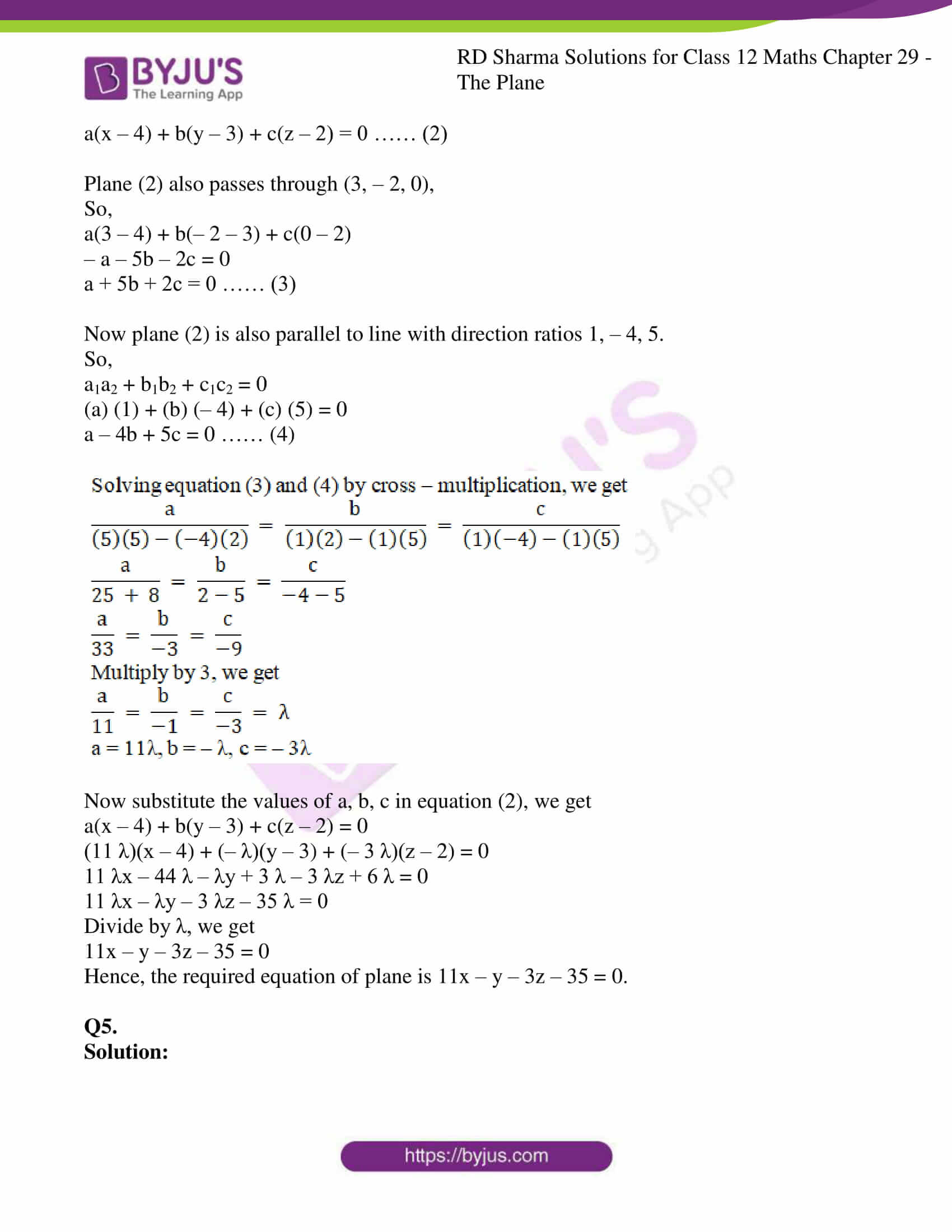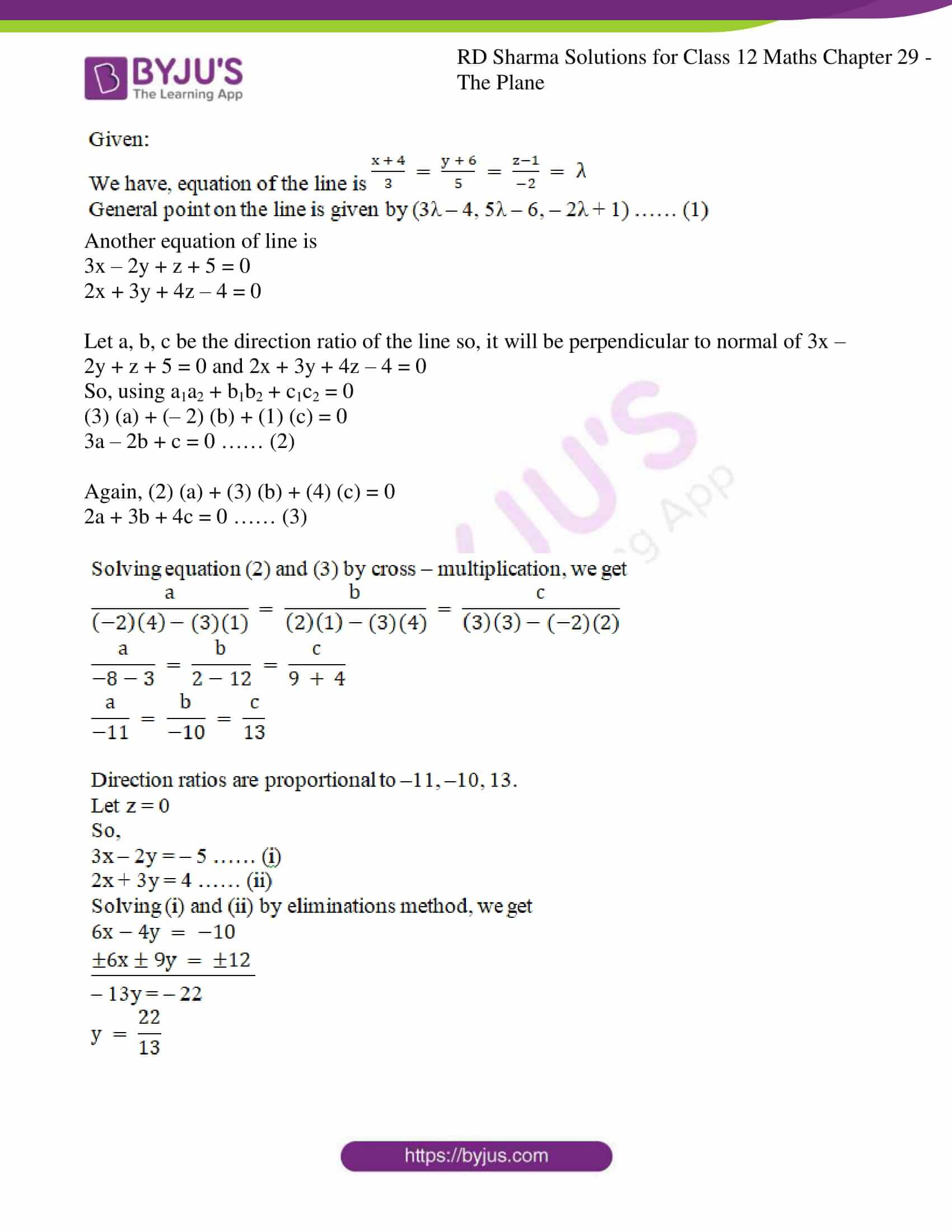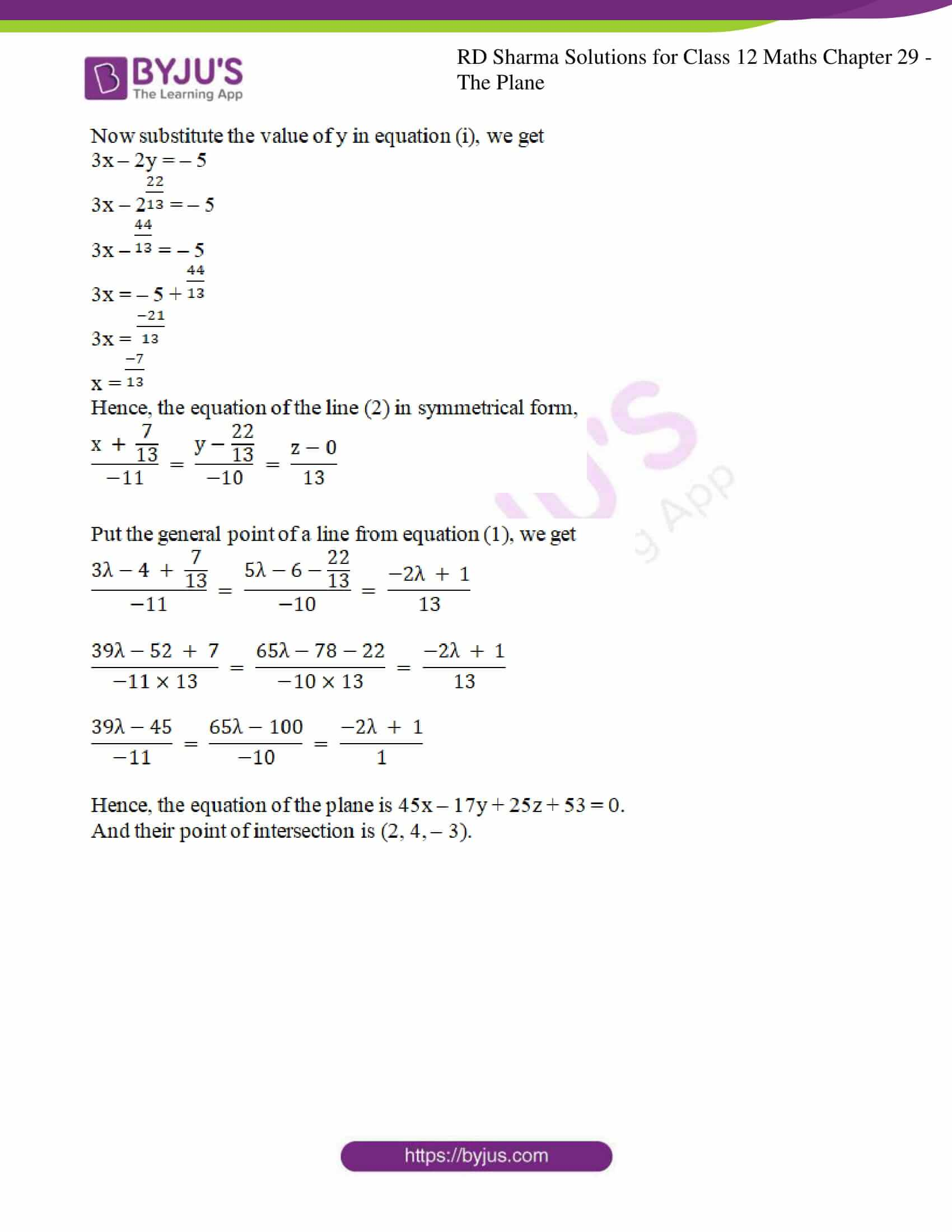### Access RD Sharma Solutions For Class 12 Maths Chapter 29 Exercise 13

EXERCISE 29.13

Q1.

Solution: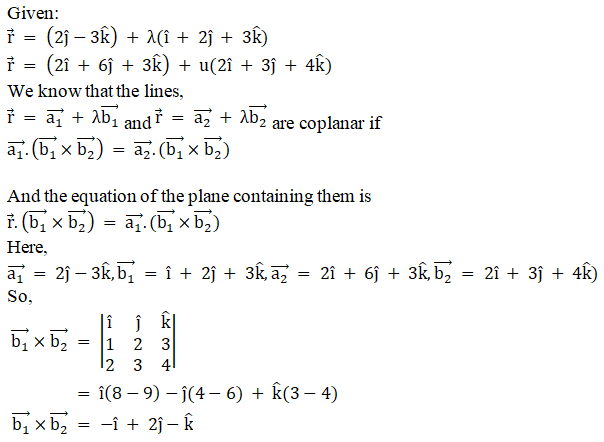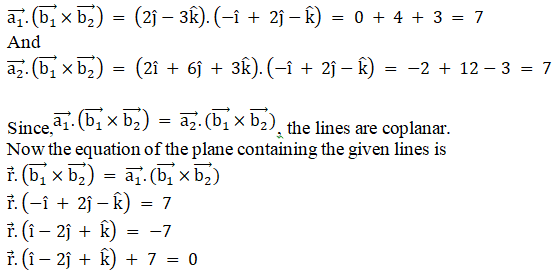Q2.

Solution: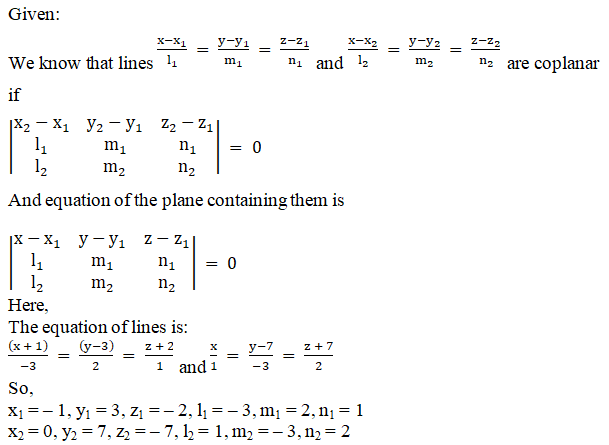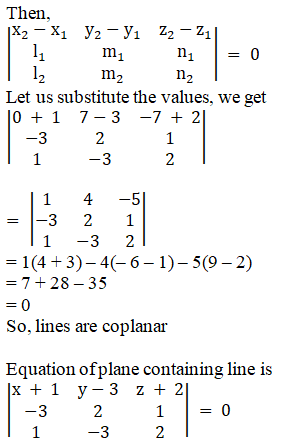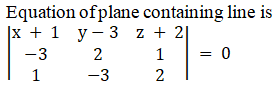(x + 1) (4 + 3) – (y – 3) (– 6 – 1) + (z + 2) (9 – 2) = 0

7x + 7 + 7y – 21 + 7z + 14 = 0

7x + 7y + 7z = 0

Divide by 7, we get

x + y + z = 0

Hence, the required equation of plane is x + y + z = 0.

Q3.

Solution: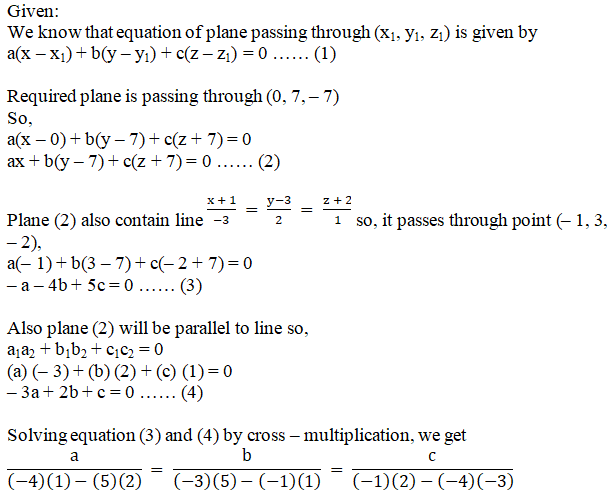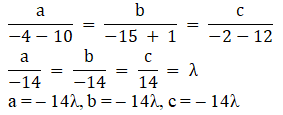Substitute a, b, c in equation (2), we get

ax + b(y – 7) + c(z + 7) = 0

(– 14λ)x + (– 14λ) (y – 7) + (– 14λ) (z + 7) = 0

Dividing by (– 14λ) we get

x + y – 7 + z + 7 = 0

x + y + z = 0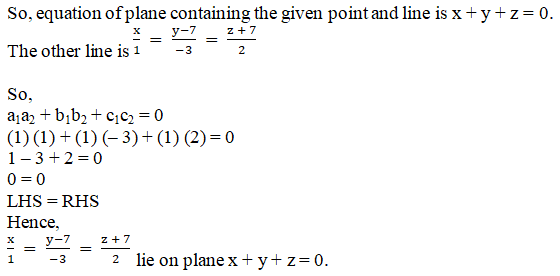Q4.

Solution:

Given:

We know that equation of plane passing through (x1, y1, z1) is given by

a(x – x1) + b(y – y1) + c(z – z1) = 0 …… (1)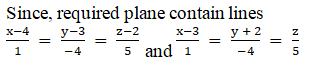Thus, required plane passes through points (4, 3, 2) and (3, – 2, 0).

So equation of required plane is

a(x – 4) + b(y – 3) + c(z – 2) = 0 …… (2)

Plane (2) also passes through (3, – 2, 0),

So,

a(3 – 4) + b(– 2 – 3) + c(0 – 2)

– a – 5b – 2c = 0

a + 5b + 2c = 0 …… (3)

Now plane (2) is also parallel to line with direction ratios 1, – 4, 5.

So,

a1a2 + b1b2 + c1c2 = 0

(a) (1) + (b) (– 4) + (c) (5) = 0

a – 4b + 5c = 0 …… (4)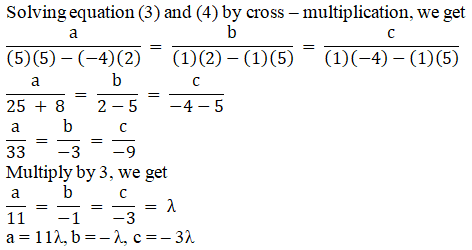Now substitute the values of a, b, c in equation (2), we get

a(x – 4) + b(y – 3) + c(z – 2) = 0

(11 λ)(x – 4) + (– λ)(y – 3) + (– 3 λ)(z – 2) = 0

11 λx – 44 λ – λy + 3 λ – 3 λz + 6 λ = 0

11 λx – λy – 3 λz – 35 λ = 0

Divide by λ, we get

11x – y – 3z – 35 = 0

Hence, the required equation of plane is 11x – y – 3z – 35 = 0.

Q5.

Solution: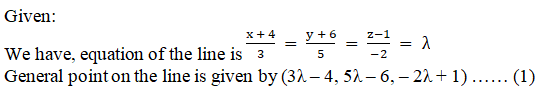Another equation of line is

3x – 2y + z + 5 = 0

2x + 3y + 4z – 4 = 0

Let a, b, c be the direction ratio of the line so, it will be perpendicular to normal of 3x – 2y + z + 5 = 0 and 2x + 3y + 4z – 4 = 0

So, using a1a2 + b1b2 + c1c2 = 0

(3) (a) + (– 2) (b) + (1) (c) = 0

3a – 2b + c = 0 …… (2)

Again, (2) (a) + (3) (b) + (4) (c) = 0

2a + 3b + 4c = 0 …… (3)